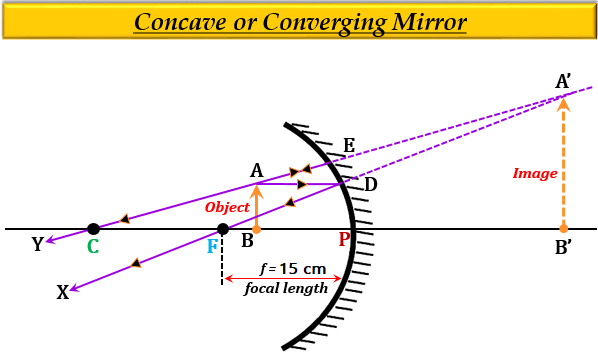# We wish to obtain an erect image of an object, using a concave mirror of focal length 15 cm. What should be the range of distance of the object from the mirror? What is the nature of the image? Is the image larger or smaller than the object? Draw a ray diagram to show the image formation in this case.

Range of distance of the object from the concave mirror to obtain an erect image:
To obtain an erect image of an object, using a concave mirror of a focal length of 15 cm, the range of the object distance placed in front of the mirror is from 0 to 15 cm. This is because in a concave mirror erect image is formed only when the object is placed between the pole  $(P)$ and the focus $(F)$. Therefore, the object distance should be less than 15 cm.

Nature of the image formed by the concave mirror of focal length 15 cm:

The nature of the image formed will be magnified.

Size of the image:
The size of the image formed by a concave mirror will be larger than the size of the object.

Ray diagram to show the image formation by a concave mirror

The ray diagram of the image formation by a concave mirror of 15 cm focal length is shown below:
An object AB is placed between the range $(0\ to\ 15\ cm)$ and an erect, magnified image A'B' is formed.Updated on: 10-Oct-2022

172 Views